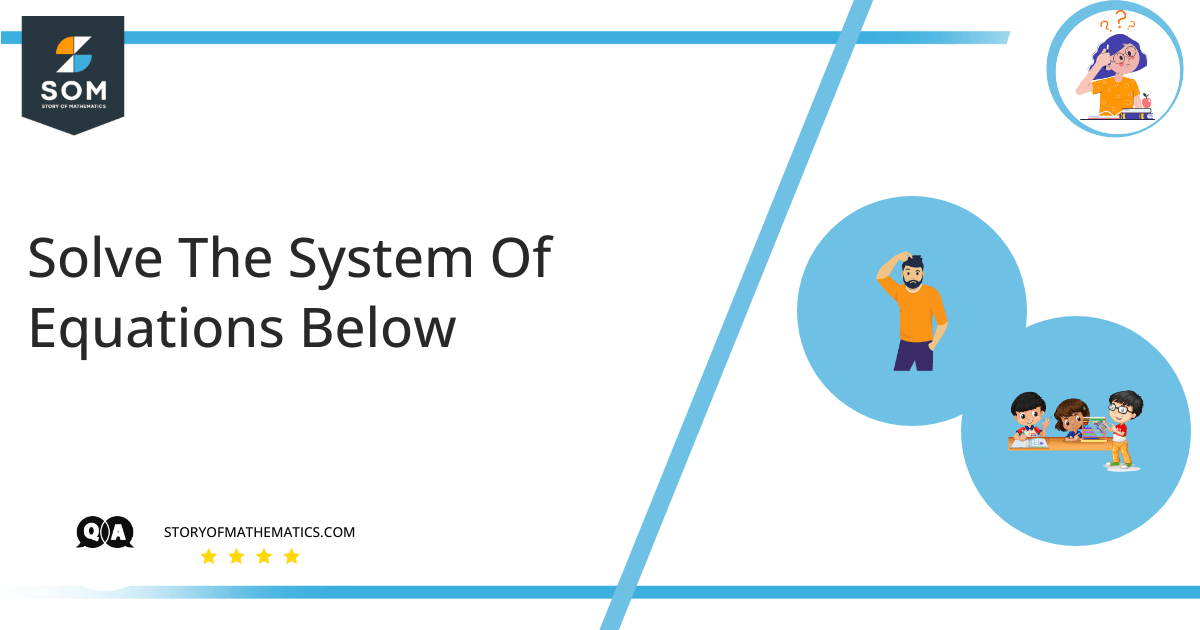# Solve the system of equations below.\begin{align}& 2x+3y=7\\& y=-x+3\end{align}

In this question, a system of two equations is given. We are required to find the solution to the given system.

A set or collection of simultaneous linear or non-linear equations is called a system of equations. This set or collection is finite and usually has common solutions. A system of equations can be categorized in the same way that a single equation can. The solution of the equation system entails determining the values of the variables present in the set of equations. We calculate the unknown values of the variables while keeping the equations on each side balanced. The values of the variables which can be found by solving the system of equations should satisfy the equations.

A system of equations is said to have a consistent solution if all the variables have a unique value, otherwise, it is said to be inconsistent. A matrix with elements as the coefficients of the linear equation can be used to represent the system of equations.  A system with two equations can be solved using the substitution technique and the systems with more than two equations can be solved using matrices.

Defined the given equations as:

$2x+3y=7$                            (1)

$y=-x+3$                               (2)

Using the substitution technique, substitute the value of $y$ from equation (2) in (1) as:

$2x+3(-x+3)=7$

$2x-3x+9=7$

$-x=7-9$

$-x=-2$

$x=2$

Now, substitute the value of $x$ back in (2) so we get:

$y=-(2)+3$

$y=1$

Now substitute the values of $x$ and $y$ back in the given equations to see if they satisfy both.

For equation (1):

$2(2)+3(1)=7$

which is satisfied.

For equation (2):

$1=-2+3$

which is also satisfied.

Hence, the given equation has a solution $(2,1)$.## Alternative Solution

Now we use the method of elimination to find the solution to the given equations. Since:

$2x+3y=7$                            (1)

$y=-x+3$                               (2)

Rearrange (2) as:

$x+y=3$                                (3)

Next, multiply (3) by $2$ and subtract (3) from (2) as:

$2x+3y=7$

$\underline{\pm\,2x\pm\,2y=\pm\,6}$

$y=1$

Again, substitute $y$ in (3) to get $x$ as:

$x+1=3$

$x=3-1$

$x=2$

So, from both methods, the result is the same.

## Example

Use the elimination method to solve the following system of equations.

$-2x+y=14$

$x+3y=7$

### Solution

Define the equations as:

$-2x+y=14$                           (1)

$x+3y=7$                               (2)

First, eliminate $x$. For this purpose, multiply equation (2) by $2$ and then add both the equations.

$-2x+y=14$

$\underline{2x+6y=14}$

$7y=28$

$y=4$

Substitute $y$ back in equation (2) to get the value of $x$ as:

$x+3(4)=7$

$x+12=7$

$x=7-12$

$x=-5$

Hence the solution is $(-5,4)$.